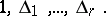# Jacobi method

(diff) ← Older revision | Latest revision (diff) | Newer revision → (diff)

A method for reducing a quadratic form (cf. also Quadratic forms, reduction of) to canonical form by using a triangular transformation of the unknowns; it was suggested by C.G.J. Jacobi (1834) (see ).

Let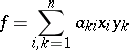be a given bilinear form (not necessarily symmetric) over a field. Suppose that its matrix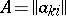satisfies the condition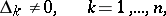(1)

where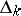is the minor of orderin the upper left-hand corner. Thencan be written in the form(2)

where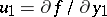,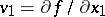, and for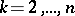,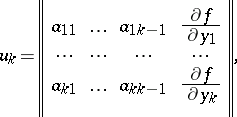(3a)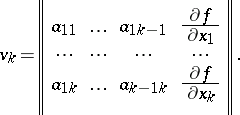(3b)

In particular, ifis a symmetric matrix satisfying (1) andis the quadratic form with matrix, thencan be reduced to the canonical form(4)

by using the following transformation of the unknowns: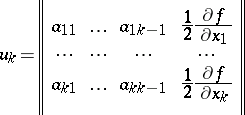(5)

for, and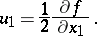This transformation has a triangular matrix, and can be written as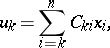(6)

where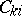is the minor ofthat stands in the rows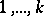, and in the columns.

The formulas (2)–(7) are called Jacobi's formulas.

When the matrix ofsatisfies only the conditions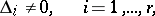whereis the rank of the form,can be reduced to the canonical form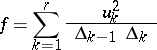(7)

(here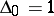) by a triangular transformation of the unknowns. This reduction can be realized by using the Gauss method (see ). If, in particular,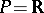, then the positive index of inertia ofis equal to the number of preservations of sign, and the negative index of inertia is equal to the number of changes of sign in the series of numbers